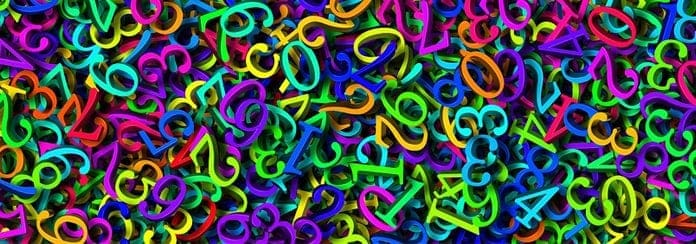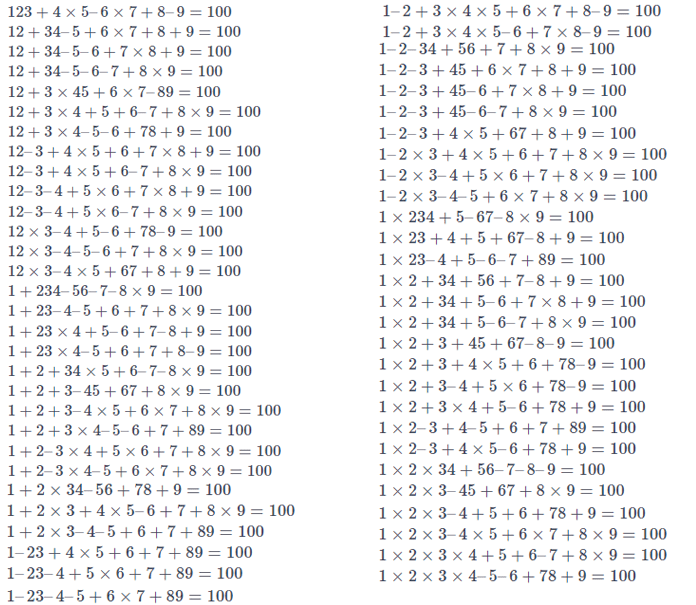# How to Get 100 With Nine Different FiguresThere are a lot of different math tricks that can impress you with their beauty and compactness. In this post, you will find not only their examples but also will try to create your own. To begin with, a few “Mathematical Curiosities” are introduced by the famous Russian mathematician and physicist Yakov Perelman (1882-1942) in his popular book “Arithmetic for entertainment”. Here are some of them:

$1 + 2 + 3 + 4 + 5 + 6 + 7 + 8 × 9 = 100$
$12 + 3 – 4 + 5 + 67 + 8 + 9 = 100$
$123 – 45 – 67 + 89 = 100$
$24 \frac{3}{6} + 75 \frac{9}{18} = 100$
$94 + 157\frac{8}{263} = 100$

In each of these expressions, we obtain the number 100 by operating with the nine natural numbers from 1 to 9 in different mathematical methods . Let’s now try to do something similar, formulating the problem in the following way:

Arrange symbols of the four arithmetic operations $+, -, ×, ÷$  in a row of numbers $1, 2, 3, 4, 5, 6, 7, 8, 9$ in any place (or don’t put anything, forming a double-digit, three-digit number, etc.) in such way that the obtained value  is equal to 100. Using other characters and operations (braces, root signs, powers, etc.) is not allowed.

Here are 101 solutions to this problem, grouped by a set of the operations performed.

$123 + 45 – 67 + 8 – 9 = 100$
$123 + 4 – 5 + 67 – 89 = 100$
$123 – 45 – 67 + 89 = 100$
$123 – 4 – 5 – 6 – 7 + 8 – 9 = 100$
$12 + 3 + 4 + 5 – 6 – 7 + 89 = 100$
$12 + 3 – 4 + 5 + 67 + 8 + 9 = 100$
$12 – 3 – 4 + 5 – 6 + 7 + 89 = 100$
$1 + 23 – 4 + 56 + 7 + 8 + 9 = 100$
$1 + 23 – 4 + 5 + 6 + 78 – 9 = 100$
$1 + 2 + 34 – 5 + 67 – 8 + 9 = 100$
$1 + 2 + 3 – 4 + 5 + 6 + 78 + 9 = 100$

$12 + 34 + 5 × 6 + 7 + 8 + 9 = 100\\12 + 3 × 4 + 5 + 6 + 7 × 8 + 9 = 100$
$1 + 2 + 3 + 4 + 5 + 6 + 7 + 8 × 9 = 100$
$1 + 2 × 3 + 4 + 5 + 67 + 8 + 9 = 100$
$1 × 2 + 34 + 5 + 6 × 7 + 8 + 9 = 100$
$1 × 2 × 3 + 4 + 5 + 6 + 7 + 8 × 9 = 100$
$1 × 2 × 3 × 4 + 5 + 6 + 7 × 8 + 9 = 100$$12 + 3 + 4 – 56 ÷ 7 + 89 = 100$

$1 + 234 × 5 × 6 ÷ 78 + 9 = 100\\1 + 2 + 3 × 4 × 5 ÷ 6 + 78 + 9 = 100\\1 + 2 × 3 × 4 × 5 ÷ 6 + 7 + 8 × 9 = 100\\1 × 23 + 4 + 56 ÷ 7 × 8 + 9 = 100\\1 × 2 ÷ 3 + 4 × 5 ÷ 6 + 7 +89 = 100\\1 ÷ 2 × 3 ÷ 4 × 56 + 7 + 8 × 9 = 100\\1 ÷ 2 ÷ 3 × 456 + 7 + 8 + 9 = 100$

## Addition, subtraction, multiplication and division

$12 ÷ 3 + 4 × 5 – 6 – 7 + 89 = 100\\12 ÷ 3 + 4 × 5 × 6 – 7 – 8 – 9 = 100\\12 ÷ 3 + 4 × 5 × 6 × 7 ÷ 8 – 9 = 100\\12 ÷ 3 ÷ 4 + 5 × 6 + 78 – 9 = 100\\1 + 234 × 5 ÷ 6 – 7 – 89 = 100\\1 + 23 – 4 + 56 ÷ 7 + 8 × 9 = 100\\1 + 23 × 4 + 56 ÷ 7 + 8 – 9 = 100\\1 + 2 + 3 × 4 × 56 ÷ 7 – 8 + 9 = 100\\1 + 2 × 3 – 4 + 56 ÷ 7 + 89 = 100\\1 – 2 – 3 + 4 × 56 ÷ 7 + 8 × 9 = 100\\1 × 23 – 4 – 56 ÷ 7 + 89 = 100\\1 × 23 × 4 – 56 ÷ 7 ÷ 8 + 9 = 100\\1 × 2 + 34 – 56 ÷ 7 + 8 × 9 = 100\\1 × 2 – 3 + 4 + 56 ÷ 7 + 89 = 100\\1 ÷ 2 × 34 – 5 + 6 – 7 + 89 = 100$

These 101 ratios apparently are a comprehensive solution of the problem posed at the beginning. However, if you change the initial conditions, then, of course, other solutions are possible. Consider some of the possible cases and golve them!## Using parentheses:

$(1 + 2 – 3 – 4) × (5 – 6 – 7 – 8 – 9) = 100\\(1 ÷ 2 – 3) × 4 ÷ 5 + 6 + 7 + 89 = 100\\(1 + 2 + 3 – 4 + 5) ÷ 6 × 78 + 9 = 100\\1 × (2 + 3) × 4 × 5 × (6 – 7) × (8 – 9) =100$

## Adding a minus in front of the unit:

$–123 – 4 + 5 × 6 × 7 + 8 + 9 = 100\\–123 + 45 × 6 – 7 × 8 + 9 = 100 \\–1 ÷ 2 – 3 + 45 ÷ 6 + 7 + 89 = 100 \\–1 ÷ 2 × 34 + 5 × 6 + 78 + 9 = 100$

$(–1 + 23) × 4 ÷ 56 × 7 + 89 = 100\\((–1 × 2 ÷ 3 – 4) × 5 ÷ 6 + 7 + 8) × 9 = 100$

Despite the popularity of this problem, one can only wonder why “reverse” puzzle attracts so little attention. Under the “reverse” we mean a problem with the reverse sequence of digits 9 8 7 6 5 4 3 2 1, and the same set of conditions as in the original problem.

Give it a go, it may come quite exciting! Don’t limit yourself with the range of the four basic arithmetic operations, pick any. Try using, for example, the arithmetic sign of the square root:
$123 – 4 + 5 – 6 – 7 – 8 – \sqrt{9} = 100$
and Antje:
$123 – 4 – 5 – [6.7] – [8,9] = 100$
or factorial:
$1 + 2 + 3 + 4 + 5! – 6 – 7 – 8 – 9 = 100$
or…